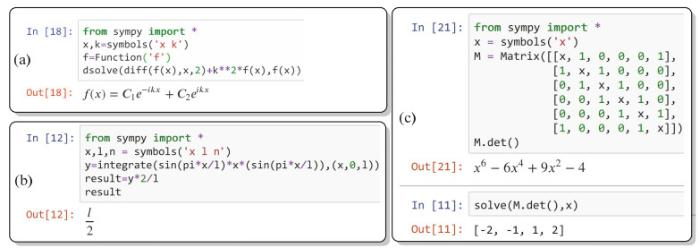## Application of SymPy in the Teaching of Structural Chemistry Course

 基金资助: 广西师范大学本科教学改革工程项目.  2019JGB32Abstract

Structural chemistry is one of the core basic courses of chemistry-related majors. In the course of teaching structural chemistry, complex formula derivation, mathematical operations and function image drawing have always been the most difficult points of the course. This article introduces the application of the Python scientific computing library SymPy for symbolic calculation and function image drawing in the teaching of structural chemistry through specific examples. The programming language involved in the library is easy to learn, understand, and operate. It can effectively help students overcome their fear to learning, deepen their understanding of structural chemistry, and also help subsequent learning of computational chemistry courses.

Keywords： Structural chemistry ; Teaching reform ; Python ; SymPy

Hou Cheng. Application of SymPy in the Teaching of Structural Chemistry Course. University Chemistry[J], 2021, 36(2): 2003046-0 doi:10.3866/PKU.DXHX202003046

## 1 SymPy库安装与使用

Sympy库是基于Python语言的开源库(图 1(a))，主要针对于科学计算过程中的符号计算，如微积分运算，微分方程求解，因式分解和矩阵运算等。它安装非常简便，对于已经安装了Python运行环境的计算机，直接在cmd命令行中运行pip install sympy命令即可完成安装。对于没有安装python环境的计算机，笔者推荐直接安装Python环境管理工具Anaconda，在该套装软件中，包含了常用的Python科学计算库，同时提供了Web交互式编程程序Jupyter Notebook以及强大的集成开发环境Spyder，特别是Jupyter Notebook仅仅通过浏览器就可以编写运行Python代码(图 1(b))，非常适合课堂教学演示，接下来我们将通过具体实例介绍其基本功能，实例运行环境为Python 3.7.4版本。

### 图1### 2.1 微分方程求解

1. from sympy import *            #导入SymPy库

2. x, k=symbols('x k')            #定义x, k为符号

3. f=Function('f')            #定义f为函数

4. dsolve(diff(f(x), x, 2)+k**2*f(x), f(x))            #dsolve函数求解微分方程

### 图2(a)微分方程求解应用；(b)积分运算应用；(c)矩阵运算应用

### 2.2 积分运算

1. from sympy import *            #导入SymPy库

2. x, l = symbols('x l')            #定义x, l, 为符号

3. n = Symbol("n", positive=True, integer=True, nonzero=True)            #定义n为符号, 且为非零正整数

4. y = integrate(sin(n*pi*x/l)*x*sin(n*pi*x/l), (x, 0, l))            #进行积分运算

5. result = y*2/l            #乘以归一化系数

6. result

### 2.3 矩阵运算和行列式展开

1. from sympy import *            #导入SymPy库

2. x = symbols('x')            #定义x为符号

3. M = Matrix([

4. [x, 1, 0, 0, 0, 1],

5. [1, x, 1, 0, 0, 0],

6. [0, 1, x, 1, 0, 0],

7. [0, 0, 1, x, 1, 0],

8. [0, 0, 0, 1, x, 1],

9. [1, 0, 0, 0, 1, x]])            #定义矩阵

10. M.det()            #行列式展开

11. solve(M.det(), x)            #求解方程

### 3.1 势箱模型波函数绘制

1. from sympy import *            #导入SymPy库

2. n=3            #给出量子数

3. x = Symbol('x')            #定义x为符号

4. plot((sqrt(2)*sin(n*pi*x)), (x, 0, 1))            #画出函数图像，x取值范围为0到1

1. from sympy import *            #导入SymPy库

2. from sympy.plotting import plot3d            #导入SymPy三维函数图像库

3. nx=3;ny=3            #给出量子数

4. x, y = symbols('x y')            #定义x, y为符号

5. f = 2*sin(nx*pi*x)*sin(ny*pi*y)            #给出波函数表达式

6. plot3d(f, (x, 0, 1), (y, 0, 1), title='2D particle in a box', xlabel='x', ylabel='y')            #画出函数图像

### 图3(a)一维势箱波函数图像绘制；(b)二维势箱波函数图像绘制

### 3.2 原子轨道波函数图像绘制

1. from sympy import Ynm, Symbol, simplify            #导入SymPy库中球谐函数

2. from sympy.abc import n, m            #导入参数

3. theta = Symbol("theta")            #定义theta为符号

4. phi = Symbol("phi")            #定义phi为符号

5. Ynm(1, 0, theta, phi).expand(func=True)            #给出n=1, m=0时的球谐函数表达式

### 图4(a)球谐函数调用实例；(b)氢原子轨道波函数调用实例；(c) 2Pz轨道波函数等高线图绘制

1. from sympy import *            #导入SymPy库

2. from sympy.physics.hydrogen import Psi_nlm            #导入SymPy库中氢原子波函数

3. Z=Symbol("Z", positive=True, integer=True, nonzero=True)            #Z为核电荷数

4. r=Symbol("r", real=True, positive=True)            #定义r为正实数

5. theta=Symbol("theta", real=True)            #定义theta为符号

6. phi=Symbol("phi", real=True)            #定义phi为符号

7. Psi_nlm(2, 1, 0, r, phi, theta, 1)            #给出n=2, l=1, m=0时的氢原子波函数表达式

1. import matplotlib.pyplot as plt            #导入matplotlib图形库

2. import numpy as np            #导入numpy库

3.

4. n = 256

5. x = np.linspace(-10, 10, n); z = np.linspace(-10, 10, n)

6. X, Z= np.meshgrid(x, z)            #生成二维网格

7. r = np.sqrt(X**2+Z**2)

8. theta = np.arctan(X/Z)

9. f = np.sqrt(2)/(8*np.sqrt(np.pi))*r*np.exp(-r/2)*np.cos(theta)            #给出函数表达式

10. plt.figure(figsize=(5, 5))

11. plt.ylabel('z'); plt.xlabel('x')

12. plt.grid(True)

13. C = plt.contour(X, Z, f, 8)            #做等高线图

14. plt.clabel(C, inline=True, fontsize=10)

15. plt.show()

### 4.1 学情分析

 索引 师生活动 教学内容 设计意图 导入课程 介绍休克尔分子轨道理论内容以及处理丁二烯电子结构的主要步骤。休克尔分子轨道理论基本假设(1) σ–π键分离；(2)积分简化具体计算步骤：以丁二烯为例(1)根据分子结构构建久期方程组(2)方程组系数行列式展开(3)求解行列式展开式中的根并得到能量的表达式(4)将不同的根依次带入求解该能级分子轨道系数 总结复习上节所学知识，为本节课内容进行铺垫。 提出问题 让学生上台黑板书写丙烯自由基的久期行列式，并尝试展开。 丙烯自由基的久期行列式为：$\left| {\begin{array}{*{20}{c}} x&1&0 \\ 1&x&1 \\ 0&1&x \end{array}} \right| = 0$展开后表达式为：${x^3} - 2x = 0$ 点评学生书写结果，指出随着所处理体系的增大，行列数阶数上升，行列式展开计算量增大。 提出解决方案 介绍SymPy矩阵操作函数和求解方程，线性方程组的solve函数。 以二阶行列式利用计算机演示行列式展开，代码如下：1. from sympy import *2. a, b, c, d = symbols('a b c d')3. M = Matrix([[a, b], [c, d]])4. M.det()运行后得到ad−bc以二元一次方程组为例，演示求解线性方程组方法，代码如下：1. from sympy import *2. x, y= symbols("x y")3. f1 = x+y-354. f2 = 2*x+4*y-945. solve([f1, f2], [x, y])运行后得到{x: 23, y: 12}，即为方程的根 以较为简单的例子，由浅入深讲述程序实现方法，重点帮助学生了解一些基本命令。 具体实例 介绍基于休克尔分子轨道理论，利用SymPy处理丙烯自由基电子结构的具体步骤。构建久期行列式并展开，代码如下：1. from sympy import *2. x= symbols('x')3. M = Matrix([[x, 1, 0], [1, x, 1], [0, 1, x]])4. M.det()利用solve函数求解方程，代码如下：solve(M.det(), x)求得方程的根为0，$\sqrt{2}，-\sqrt{2}$，由于x = (a − E)/b，分别带入即可解得三个能级的能量表达式将每个根分别带入到之前得方程组可以对应能级的波函数系数，在这里我们取x = $-\sqrt{2}$，同时考虑归一化条件，构建线性方程组，代码如下：1. c1, c2, c3 = symbols("c1 c2 c3")2. x = -sqrt(2)3. f1 = c1*x+c24. f2 = c1+c2*x+c35. f3 = c2+c3*x6. f4 = c1**2+c2**2+c3**2-17. solve([f1, f2, f3, f4], [c1, c2, c3])可以得到成键轨道波函数的系数为：c1 = 1/2，$c2 = \sqrt 2 {\rm{/}}2$，c3 = 1/2，波函数表达式为：${\psi _1} = \frac{1}{2}{\phi _1} + \frac{{\sqrt 2 }}{2}{\phi _2} + \frac{1}{2}{\phi _2}$同理可得其它轨道波函数表达式：${\psi _2} = \frac{{\sqrt 2 }}{2}{\phi _1} - \frac{{\sqrt 2 }}{2}{\phi _2}$${\psi _3} = \frac{1}{2}{\phi _1} - \frac{{\sqrt 2 }}{2}{\phi _2} + \frac{1}{2}{\phi _2}$ 通过具体实例讲述，帮助学生进一步熟悉休克尔分子轨道理论处理共轭分子的基本步骤；帮助学生掌握利用计算机处理数学问题的基本方法。 课后练习 布置作业，让学生自己编写处理丁二烯电子结构的代码，举一反三。作业：1)基于上述代码，基于休克尔分子轨道理论，编写处理1, 3-丁二烯的小程序，并和之前书本例题结果进行核对。2)进一步编写求1，3-丁二烯原子电荷，键级，自由价的小程序。 让学生对所学到的知识进行巩固。

### 4.2 教学目标设计

a) 掌握休克尔分子轨道内容实质，具体运算步骤；

b) 掌握SymPy在矩阵运算，求解线性方程组等数学问题的具体方法；

c) 提高学生学习兴趣，培养举一反三的能力。

#### 4.3.1 内容纲要

2) 复习休克尔分子轨道基本内容以及处理丁二烯电子结构的方法；

2) 介绍SymPy中矩阵运算，线性方程组求解方法；

2) 基于休克尔分子轨道理论，利用SymPy处理丙烯自由基分子的电子结构。

#### 4.3.2 教学重点

a) 休克尔分子轨道理论；

b) SymPy处理该问题的具体方法。

a) 板书标示教学法；

b) 迁移教学法。

## 参考文献 原文顺序 文献年度倒序 文中引用次数倒序 被引期刊影响因子

/

 〈〉